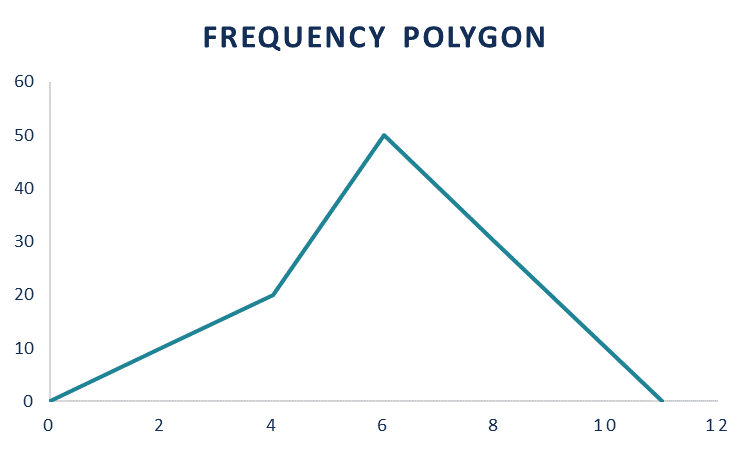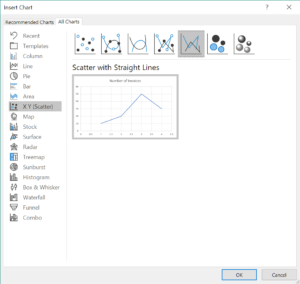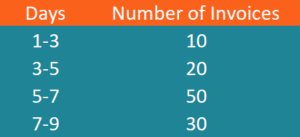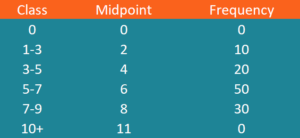# Frequency Polygon

A visual representation of a distribution

## What is a Frequency Polygon?

A frequency polygon is a visual representation of a distribution. The visualization tool is used to understand the shape of a distribution. Essentially, the frequency polygon indicates the number of occurrences for each distinct class in the dataset. In addition, the graph may be used to show the cumulative frequency distribution.The frequency polygon is a curve that is drawn on the x-axis and the y-axis. The x-axis represents the values in the dataset while the y-axis shows the number of occurrences of each distinct category.

The frequency polygon can serve as an alternative to the histogram. Both visual representations perfectly reflect the shape of a distribution. However, unlike the histogram, the frequency polygon can be easily utilized to compare multiple distributions on the same graph. In some cases, histogram and frequency polygon can be used simultaneously to get a more accurate picture of the distribution shape.

### How to Create a Frequency Polygon in Excel?

Excel can be a convenient and simple tool to create the frequency polygon of a distribution. The frequency polygon can be created using the following steps:

1. Determine the classes in the dataset by defining the lower and upper limits of each class and arrange them in one column.
2. Identify the midpoints of each class. The midpoints can be found using the formula below:

Midpoint = (Lower limit + Upper limit) / 2

The identified midpoints must be arranged in a separate column.

1. Calculate the frequencies for each class and arrange them in a separate column.
2. To ensure that our graph is truly a polygon (i.e., the graph is closed-shape), we must include the first and last class with zero frequencies.
3. Highlight the column that contains the midpoints for each class and the column that contains the frequencies.
4. Select Insert -> Charts -> Insert Scatter -> Scatter with Straight Lines.### Example of Frequency Polygon

You are a financial analyst in a retail business. You are preparing a report regarding the current financial conditions of the company. One part of the report describes the management of the company’s accounts payable. You obtain the data that defines how many days does is required to settle each invoice.You need to create a frequency polygon that will reflect the distribution of the accounts payable. Using the data from the table above, let’s create the frequency polygon:

1. The classes within the dataset are listed in the first column on the table above.

2. The midpoints for each class can be calculated in the following way:

Midpoint (1-3) = (1 + 3) / 2 = 2

Midpoint (3-5) = (3 + 5) / 2 = 4

Midpoint (5-7) = (5 + 7) / 2 = 6

Midpoint (7-9) = (7 + 9) / 2 = 8

3. The frequencies for each class are listed in the second column on the table above.

4. To ensure that our graph is closed shape, you must determine the first and last class with zero frequencies. The first class is zero days with zero frequency. The last class is 10-12 days (it must show a similar spread as the other classes) and zero frequency.

5. The input table for the creation of the frequency polygon is summarized below:1. Select the columns Mid-Point and Frequency. Then, select Insert -> Charts -> Insert Scatter -> Scatter with Straight Lines. The frequency polygon must look like the graph at the top of this article.